CAT  >  Test Level 3: Data Sufficiency - 1

# Test Level 3: Data Sufficiency - 1

Test Description

## 10 Questions MCQ Test Level-wise Tests for CAT | Test Level 3: Data Sufficiency - 1

Test Level 3: Data Sufficiency - 1 for CAT 2023 is part of Level-wise Tests for CAT preparation. The Test Level 3: Data Sufficiency - 1 questions and answers have been prepared according to the CAT exam syllabus.The Test Level 3: Data Sufficiency - 1 MCQs are made for CAT 2023 Exam. Find important definitions, questions, notes, meanings, examples, exercises, MCQs and online tests for Test Level 3: Data Sufficiency - 1 below.
Solutions of Test Level 3: Data Sufficiency - 1 questions in English are available as part of our Level-wise Tests for CAT for CAT & Test Level 3: Data Sufficiency - 1 solutions in Hindi for Level-wise Tests for CAT course. Download more important topics, notes, lectures and mock test series for CAT Exam by signing up for free. Attempt Test Level 3: Data Sufficiency - 1 | 10 questions in 20 minutes | Mock test for CAT preparation | Free important questions MCQ to study Level-wise Tests for CAT for CAT Exam | Download free PDF with solutions
 1 Crore+ students have signed up on EduRev. Have you?
Test Level 3: Data Sufficiency - 1 - Question 1

Detailed Solution for Test Level 3: Data Sufficiency - 1 - Question 1

From statement I alone, we cannot figure out the position of A with respect to D, as D could be to the north-east, south-east, or east of A.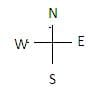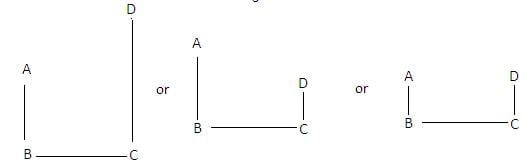So, I alone is not sufficient.
From II alone, we cannot figure out the direction of A with respect to D since A could be to the east or west of D.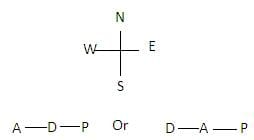So II alone is not sufficient.
From I and II together, we can figure out the direction of A with respect to D.
So, both I and II are sufficient to answer the question.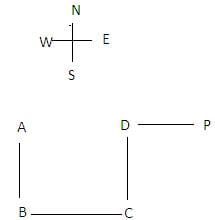Test Level 3: Data Sufficiency - 1 - Question 2

Detailed Solution for Test Level 3: Data Sufficiency - 1 - Question 2

Average score of all 5 = 75 ÷ 5 = 15, which is the score of R.
So, score of R is 15.
Now, from I alone, we know that sum of scores of S and T is 30 and that of Q and P is 30. So, S and T or Q and P could have scores of 14 and 16 or 10 and 20 or vice-versa.
Thus, no definite conclusion is drawn.
Thus, statement I alone isn't enough.
From II alone, sum of scores of Q and S is 24 and only combination that is possible is 10 and 14. thus 16 and 20 are scores of P and T. Since P scored more than T, thus score of P is 20, which is the highest among of the group.
Thus, statement II alone is sufficient to answer the question, but statement I alone is not sufficient to answer the question.

Test Level 3: Data Sufficiency - 1 - Question 3

### Directions: The following problem consists of a question and three statements (I), (II) and (III) given below it. You have to find out which of the statements is/are redundant for determining the answer to the given question or can be dispensed with. A, B, C, D, E, F and G are sitting on a single bench facing North. C is second to the left of A. C is not the immediate neighbour of E. Q. Who is sitting exactly in the middle? I. G is second to the left of F, who is not an immediate neighbour of C. II. E is the immediate neighbour of A. III. B is the immediate neighbour of G and F.

Detailed Solution for Test Level 3: Data Sufficiency - 1 - Question 3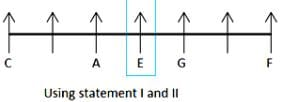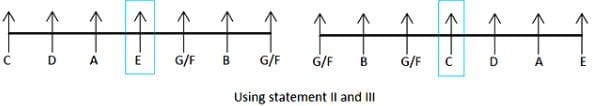Using statements I and III, we cannot make particular arrangement as there are many possibilities.
Using statements I and II, we can determine who is sitting in the middle.
It means we can find answer using I and II, so statement III is redundant.

Test Level 3: Data Sufficiency - 1 - Question 4

Directions: In the following problem, a question followed by three statements (I), (II) and (III) is given. You have to determine which statement(s) is/are sufficient/necessary to answer the question.
Five students participated in a race wearing t-shirts of different colours i.e. black, white, yellow, red and grey. Their names are Zora, Ajay, Nancy, Mani and Preet. Nancy finished the race second. The student wearing red t-shirt finished the race fourth.

Q. What is the colour of the t-shirt, which Preet was wearing during the race?
I. Student wearing white t-shirt finished the race immediately after the student wearing yellow t-shirt. Mani finished the race immediately after the one wearing black t-shirt.
II. One, who finished the race at first place was not wearing grey t-shirt. The one who stood third was not wearing yellow t-shirt.
III. Two persons finished the race between Zora and Mani. Preet finished the race immediately after the student wearing white t-shirt.

Detailed Solution for Test Level 3: Data Sufficiency - 1 - Question 4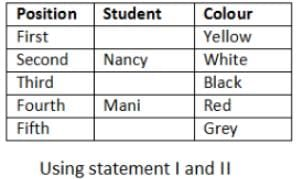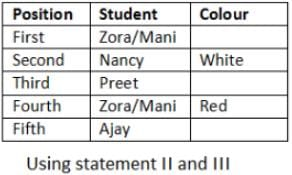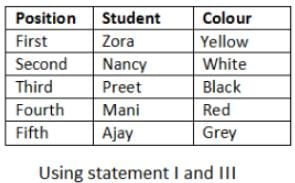The question can be answered using statements I and III.

Test Level 3: Data Sufficiency - 1 - Question 5

Directions: In the following problem, a question followed by three statements (I), (II) and (III) is given. You have to determine which statement(s) is/are sufficient/necessary to answer the question.
A is 10 m to the South of D, who is 5 m to the West of R.

Q. What is the position of T with respect to S?
I. Z is 7 m to the North of D. T is 10 m to the South of R.
II. S is 5 m to the East of R, who is 10 m to the South of F.
III. F is 20 m to the North of T. S is 10 m to the North of Q.

Detailed Solution for Test Level 3: Data Sufficiency - 1 - Question 5

We can answer the question using statement II and either I or III.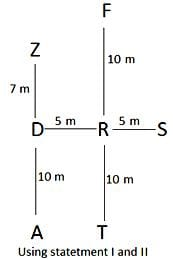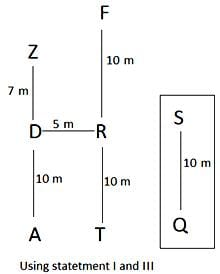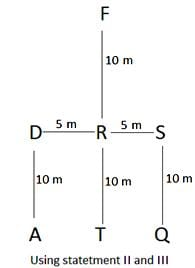Test Level 3: Data Sufficiency - 1 - Question 6

Directions: In the following problem, a question followed by three statements (I), (II) and (III) is given. You have to determine which statement(s) is/are sufficient/necessary to answer the question.
A 7 storey building has the ground floor marked as first floor and top floor marked as seventh floor. Seven persons live on different floors of the building. U lives on sixth floor.
Q. Who lives on the top floor?
I. R lives on even numbered floor and P lives on the floor which is three floors above the floor of R.
II. S is living on the floor, which is immediately below the floor of P. Q lives on a floor, which is above the floor of S.
III. R lives on the floor, which is two floors below the floor of S. T does not live on any floor above the floor of R.

Detailed Solution for Test Level 3: Data Sufficiency - 1 - Question 6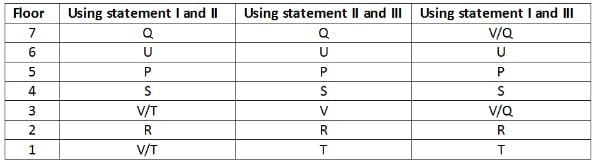Using statement II and either I or III, question can be answered.

Test Level 3: Data Sufficiency - 1 - Question 7

Directions: The following question is accompanied by statements (I), (II) and (III). You have to determine which statement(s) is/are sufficient/necessary to answer the question.
Friends M, N, O, P, Q, R, S and T are sitting around a circular table at equal distances. Some of them are facing centre and some are facing outwards. T is facing centre and Q is third to the left of T. N is not the immediate neighbour of Q.
Q. Is O sitting on the immediate left of Q?
I. S is third to the left of N. O is the immediate neighbour of Q.
II. O and P are sitting fourth to each other. N is the neighbour of P.
III. P is third to the right of Q. R and M are sitting fourth to each other.

Detailed Solution for Test Level 3: Data Sufficiency - 1 - Question 7

Using statements II and III, we get to know whether Q is facing centre or outwards and also the position of O.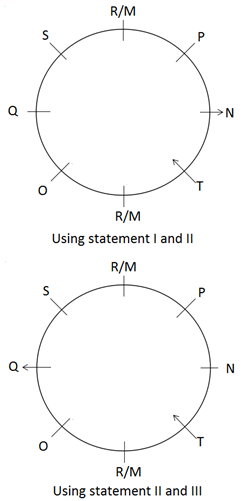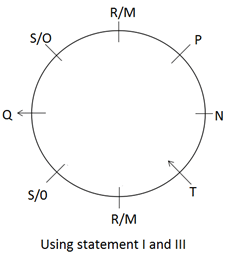Test Level 3: Data Sufficiency - 1 - Question 8

Directions: In the following problem, a question followed by three statements (I), (II) and (III) is given. You have to determine which statement(s) is/are sufficient/necessary to answer the question.
D is 10 m to the West of F, which is 15 m to the North of S.Q. In which direction is R with respect to F?I.
Q is 5 m to the South of D. Z is 5 m to the West of Q.
II. Q is 10 m to the North of Y, which is 15 m to the West of T.
III. Z is 20 m to the West of R.

Detailed Solution for Test Level 3: Data Sufficiency - 1 - Question 8

Using statements I and III only, we get that R is to the Southeast of F.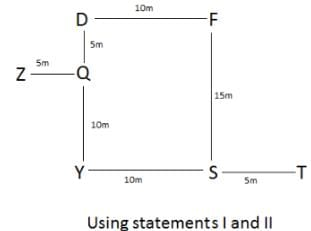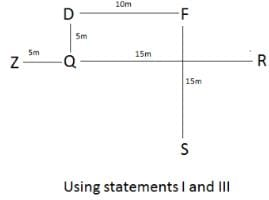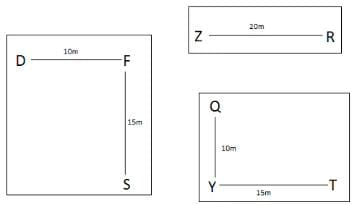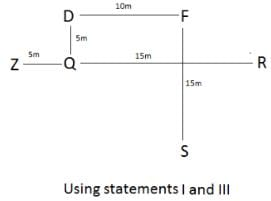Test Level 3: Data Sufficiency - 1 - Question 9

Directions: The question given below is followed by three statements (I), (II) and (III). You have to determine which of the statements is/are sufficient/necessary to answer the question.
Eight persons Ny, Xo, Ta, Le, Pi, Si, Ki and Ro are sitting around a circular table at equal distances facing the centre, but not necessarily in the same order. Ta is third to the left of Xo, who is the immediate right neighbour of Ro.
Q. What is the position of Ta with respect to Si?
I. Ny is sitting opposite to Pi.
II. Ki is third to the right of Si.
III. Le and Ki are immediate neighbours.

Detailed Solution for Test Level 3: Data Sufficiency - 1 - Question 9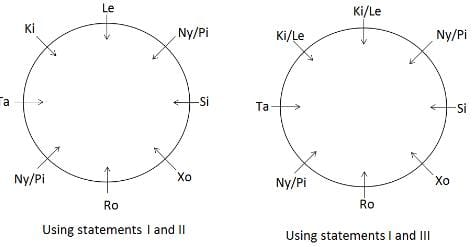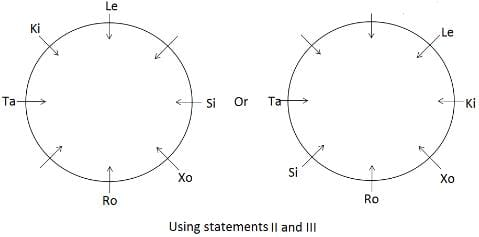Statements I and either II or III are required to answer the question.

Test Level 3: Data Sufficiency - 1 - Question 10

Directions: The following question is accompanied by three statements (I), (II) and (III). You have to determine which statements are sufficient/necessary to answer the question.
If D walks 10 m towards north then takes right turn and walks another 20 m, then he will meet F.
Q. In which direction is F with respect to X?
I. If F walks 20 m towards south then takes right turn, then he will meet G at the distance of 20 m. G is 30 m to the west of L.
II. If W walks 10 m towards west, takes right turn and walks 10 m more and then takes left turn, then he will meet F at the distance of 20 m.
III. X is 10 m to the south of W and 10 m to the east of K, who is 10 m to the east of L.

Detailed Solution for Test Level 3: Data Sufficiency - 1 - Question 10

Statements III and either I or II are required to answer the question.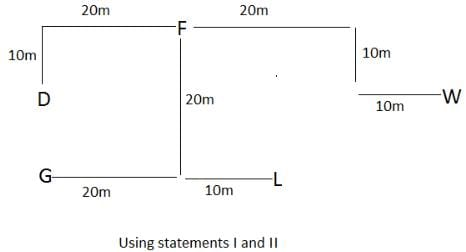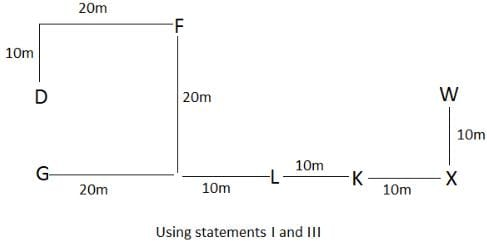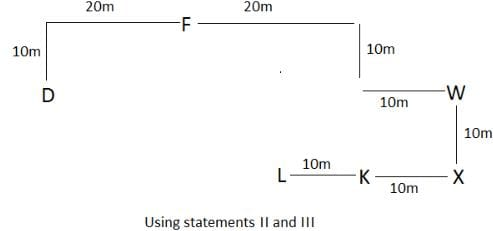## Level-wise Tests for CAT

5 docs|272 tests
 Use Code STAYHOME200 and get INR 200 additional OFF Use Coupon Code
Information about Test Level 3: Data Sufficiency - 1 Page
In this test you can find the Exam questions for Test Level 3: Data Sufficiency - 1 solved & explained in the simplest way possible. Besides giving Questions and answers for Test Level 3: Data Sufficiency - 1, EduRev gives you an ample number of Online tests for practice

## Level-wise Tests for CAT

5 docs|272 tests

### How to Prepare for CAT

Read our guide to prepare for CAT which is created by Toppers & the best Teachers